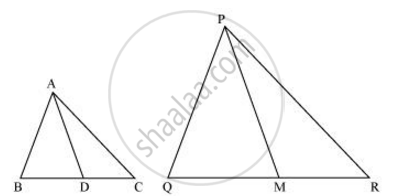# If AD and PM are medians of triangles ABC and PQR, respectively where Δ ABC ~ Δ PQR, prove that (AB)/(PQ) = (AD)/(PM) - Mathematics

Sum

AD and PM are medians of triangles ABC and PQR, respectively where Δ ABC ~ Δ PQR, prove that ("AB")/("PQ") = ("AD")/("PM")

#### SolutionIt is given that ΔABC ∼ ΔPQR

We know that the corresponding sides of similar triangles are in proportion.

:.("AB")/("PQ")=("AC")/"AD" and =("BC")/("QR")....(1)

Also, ∠A = ∠P, ∠B = ∠Q, ∠C = ∠R … (2)

Since AD and PM are medians, they will divide their opposite sides.

:."BD" = ("BC")/2  and "QM" = "QR"/2...(3)

From equations (1) and (3), we obtain

("AB")/("PQ") = ("BD")/("QM") ....(4)

In ΔABD and ΔPQM,

∠B = ∠Q [Using equation (2)]

("AB")/("PQ") =("BD")/("QM")

∴ ΔABD ∼ ΔPQM (By SAS similarity criterion)

=> ("AB")/("PQ") = ("BD")/("QM") = ("AD")/("PM")

Concept: Criteria for Similarity of Triangles
Is there an error in this question or solution?

#### APPEARS IN

NCERT Class 10 Maths
Chapter 6 Triangles
Exercise 6.3 | Q 16 | Page 141